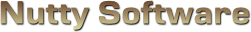Screen Space Ambient Occlusion (SSAO)

Sorry, it appears you don't have support for WebGL.

This WebGL demo requires the OES_texture_float extension to work, which your system does not support. Please ensure you are using the latest video card drivers and browser version to run this demo. If your system is up-to-date, then it is possible your video card does not support this feature.

In order to run this demo, you must meet the following requirements.

• You have a WebGL compatible video card with the latest drivers.
• Your video card is not blacklisted. You can check the current blacklist on Khronos.

Some browsers may require additional configuration in order to get WebGL to run. If you are having problems running this demo, visit the following sites.

Use mouse.

Enable SSAO

#### Attenuation

							
/// <summary>
/// </summary>

/// <summary>
/// Material source structure.
/// <summary>
struct MaterialSource
{
vec3 Ambient;
vec4 Diffuse;
vec3 Specular;
float Shininess;
vec2 TextureOffset;
vec2 TextureScale;
};

/// <summary>
/// Attributes.
/// <summary>
attribute vec3 Vertex;
attribute vec2 Uv;
attribute vec3 Normal;

/// <summary>
/// Uniform variables.
/// <summary>
uniform mat4 ProjectionMatrix;
uniform mat4 ViewMatrix;
uniform mat4 ModelMatrix;
uniform vec3 ModelScale;

uniform MaterialSource Material;

/// <summary>
/// Varying variables.
/// <summary>
varying vec4 vWorldVertex;
varying vec3 vWorldNormal;
varying vec2 vUv;
varying vec3 vViewVec;

/// <summary>
/// <summary>
void main ()
{
// Transform the vertex
vWorldVertex = ModelMatrix * vec4(Vertex * ModelScale, 1.0);
vec4 viewVertex = ViewMatrix * vWorldVertex;
gl_Position = ProjectionMatrix * viewVertex;

// Setup the UV coordinates
vUv = Material.TextureOffset + (Uv * Material.TextureScale);

// Rotate normal
vWorldNormal = normalize(mat3(ModelMatrix) * Normal);

// Calculate view vector (for specular lighting)
vViewVec = normalize(-viewVertex.xyz);
}


							
/// <summary>
/// This vertex shader prepares the geometry for rendering to a floating point texture map.
/// </summary>

/// <summary>
/// Attributes.
/// <summary>
attribute vec3 Vertex;
attribute vec3 Normal;

/// <summary>
/// Uniform variables.
/// <summary>
uniform mat4 ProjectionMatrix;
uniform mat4 ViewMatrix;
uniform mat4 ModelMatrix;
uniform vec3 ModelScale;

/// <summary>
/// Varying variables.
/// <summary>
varying vec4 vPosition;
varying vec3 vNormal;

/// <summary>
/// </summary>
void main ()
{
// Position / vertex data
vPosition = ViewMatrix * ModelMatrix * vec4(Vertex * ModelScale, 1.0);
gl_Position = ProjectionMatrix * vPosition;

// Normal data
vNormal = mat3(ViewMatrix * ModelMatrix) * Normal;
}


							
/// <summary>
/// Vertex shader for rendering a 2D plane on the screen. The plane should be sized
/// from -1.0 to 1.0 in the x and y axis. This shader can be shared amongst multiple
/// </summary>

/// <summary>
/// Attributes.
/// <summary>
attribute vec3 Vertex;
attribute vec2 Uv;

/// <summary>
/// Varying variables.
/// <summary>
varying vec2 vUv;

/// <summary>
/// <summary>
void main ()
{
gl_Position = vec4(Vertex, 1.0);

// Flip y-axis
vUv = Uv;
}


							
/// <summary>
/// </summary>

#ifdef GL_ES
precision highp float;
#endif

/// <summary>
/// Light source structure.
/// <summary>
struct LightSource
{
int Type;
vec3 Position;
vec3 Attenuation;
vec3 Direction;
vec3 Colour;
float OuterCutoff;
float InnerCutoff;
float Exponent;
};

/// <summary>
/// Material source structure.
/// <summary>
struct MaterialSource
{
vec3 Ambient;
vec4 Diffuse;
vec3 Specular;
float Shininess;
vec2 TextureOffset;
vec2 TextureScale;
};

/// <summary>
/// Uniform variables.
/// <summary>
uniform int NumLight;
uniform LightSource Light;
uniform MaterialSource Material;
uniform sampler2D Sample0;

/// <summary>
/// Varying variables.
/// <summary>
varying vec4 vWorldVertex;
varying vec3 vWorldNormal;
varying vec2 vUv;
varying vec3 vViewVec;

/// <summary>
/// <summary>
void main ()
{
// vWorldNormal is interpolated when passed into the fragment shader.
// We need to renormalize the vector so that it stays at unit length.
vec3 normal = normalize(vWorldNormal);

vec3 colour = Material.Ambient;
for (int i = 0; i < 4; ++i)
{
if ( i >= NumLight )
break;

// Calculate diffuse term
vec3 lightVec = normalize(Light[i].Position - vWorldVertex.xyz);
float l = dot(normal, lightVec);
if ( l > 0.0 )
{
// Calculate spotlight effect
float spotlight = 1.0;
if ( Light[i].Type == 1 )
{
spotlight = max(-dot(lightVec, Light[i].Direction), 0.0);
float spotlightFade = clamp((Light[i].OuterCutoff - spotlight) / (Light[i].OuterCutoff - Light[i].InnerCutoff), 0.0, 1.0);
spotlight = pow(spotlight * spotlightFade, Light[i].Exponent);
}

// Calculate specular term
vec3 r = -normalize(reflect(lightVec, normal));
float s = pow(max(dot(r, vViewVec), 0.0), Material.Shininess);

// Calculate attenuation factor
float d = distance(vWorldVertex.xyz, Light[i].Position);
float a = 1.0 / (Light[i].Attenuation.x + (Light[i].Attenuation.y * d) + (Light[i].Attenuation.z * d * d));

colour += ((Material.Diffuse.xyz * l) + (Material.Specular * s)) * Light[i].Colour * a * spotlight;
}
}

gl_FragColor = clamp(vec4(colour, Material.Diffuse.w), 0.0, 1.0);// * texture2D(Sample0, vUv);
}


							
/// <summary>
/// This fragment shader outputs vertex data to a floating point texture map
/// </summary>

#ifdef GL_ES
precision highp float;
#endif

/// <summary>
/// Stores the (Far - Near) clip value used for calculating linear depth.
/// <summary>
uniform float LinearDepth;

/// <summary>
/// Varying variables.
/// <summary>
varying vec4 vPosition;

/// <summary>
/// </summary>
void main ()
{
// Calculate and include linear depth
float linearDepth = length(vPosition) / LinearDepth;
gl_FragColor = vec4(vPosition.x, vPosition.y, vPosition.z, linearDepth);
}


							
/// <summary>
/// This fragment shader outputs normals to a floating point texture map
/// </summary>

#ifdef GL_ES
precision highp float;
#endif

/// <summary>
/// Varying variables.
/// <summary>
varying vec3 vNormal;

/// <summary>
/// </summary>
void main ()
{
vec3 normal = normalize(vNormal);
gl_FragColor = vec4(normal.x, normal.y, normal.z, 0.0);
}


							
/// <summary>
/// This fragment shader calculates the ambient occlusion contributions for each fragment.
/// 1. View-space position buffer
/// 2. View-space normal vector buffer
/// 3. Normalmap to preturb the sampling kernel
/// </summary>

#ifdef GL_ES
precision highp float;
#endif

/// <summary>
/// Texture samples used by this shader.
/// <summary>
uniform sampler2D Sample0;	// View space position data
uniform sampler2D Sample1;	// View space normal vectors
uniform sampler2D Sample2;	// Normalmap to randomize the sampling kernel
uniform vec2 TexelSize;

/// <summary>
/// Occluder bias to minimize self-occlusion.
/// <summary>
uniform float OccluderBias;

/// <summary>
/// Specifies the size of the sampling radius.
/// <summary>

/// <summary>
/// Ambient occlusion attenuation values.
/// These parameters control the amount of AO calculated based on distance
/// to the occluders. You need to play with them to find the right balance.
///
/// .x = constant attenuation. This is useful for removing self occlusion. When
///		 set to zero or a low value, you will start to notice edges or wireframes
///		 being shown. Typically use a value between 1.0 and 3.0.
///
///	.y = linear attenuation. This provides a linear distance falloff.
/// .z = quadratic attenuation. Smoother falloff, but is not used in this shader.
/// <summary>
uniform vec2 Attenuation;

/// <summary>
/// Varying variables.
/// <summary>
varying vec2 vUv;

/// <summary>
/// Sample the ambient occlusion at the following UV coordinate.
/// <summary>
/// <param name="srcPosition">3D position of the source pixel being tested.</param>
/// <param name="srcNormal">Normal of the source pixel being tested.</param>
/// <param name="uv">UV coordinate to sample/test for ambient occlusion.</param>
/// <returns>Ambient occlusion amount.</returns>
float SamplePixels (vec3 srcPosition, vec3 srcNormal, vec2 uv)
{
// Get the 3D position of the destination pixel
vec3 dstPosition = texture2D(Sample0, uv).xyz;

// Calculate ambient occlusion amount between these two points
// It is simular to diffuse lighting. Objects directly above the fragment cast
// the hardest shadow and objects closer to the horizon have minimal effect.
vec3 positionVec = dstPosition - srcPosition;
float intensity = max(dot(normalize(positionVec), srcNormal) - OccluderBias, 0.0);

// Attenuate the occlusion, similar to how you attenuate a light source.
// The further the distance between points, the less effect AO has on the fragment.
float dist = length(positionVec);
float attenuation = 1.0 / (Attenuation.x + (Attenuation.y * dist));

return intensity * attenuation;
}

/// <summary>
/// <summary>
void main ()
{
// Get position and normal vector for this fragment
vec3 srcPosition = texture2D(Sample0, vUv).xyz;
vec3 srcNormal = texture2D(Sample1, vUv).xyz;
vec2 randVec = normalize(texture2D(Sample2, vUv).xy * 2.0 - 1.0);
float srcDepth = texture2D(Sample0, vUv).w;

// The following variable specifies how many pixels we skip over after each
// iteration in the ambient occlusion loop. We can't sample every pixel within
// the sphere of influence because that's too slow. We only need to sample
// some random pixels nearby to apprxomate the solution.
//
// Pixels far off in the distance will not sample as many pixels as those close up.

// Sample neighbouring pixels
vec2 kernel;
kernel = vec2(0.0, 1.0);	// top
kernel = vec2(1.0, 0.0);	// right
kernel = vec2(0.0, -1.0);	// bottom
kernel = vec2(-1.0, 0.0);	// left

const float Sin45 = 0.707107;	// 45 degrees = sin(PI / 4)

// Sample from 16 pixels, which should be enough to appromixate a result. You can
// sample from more pixels, but it comes at the cost of performance.
float occlusion = 0.0;
for (int i = 0; i < 4; ++i)
{
vec2 k1 = reflect(kernel[i], randVec);
vec2 k2 = vec2(k1.x * Sin45 - k1.y * Sin45,
k1.x * Sin45 + k1.y * Sin45);
k1 *= TexelSize;
k2 *= TexelSize;

occlusion += SamplePixels(srcPosition, srcNormal, vUv + k1 * kernelRadius);
occlusion += SamplePixels(srcPosition, srcNormal, vUv + k2 * kernelRadius * 0.75);
occlusion += SamplePixels(srcPosition, srcNormal, vUv + k1 * kernelRadius * 0.5);
occlusion += SamplePixels(srcPosition, srcNormal, vUv + k2 * kernelRadius * 0.25);
}

// Average and clamp ambient occlusion
occlusion /= 16.0;
occlusion = clamp(occlusion, 0.0, 1.0);

gl_FragColor.x = occlusion;
}


							
/// <summary>
/// This fragment shader blends an ambient occlusion map with the rendered scene.
/// </summary>

#ifdef GL_ES
precision highp float;
#endif

/// <summary>
/// Texture samples used by this shader.
/// <summary>
uniform sampler2D Sample0;	// Rendered scene texture
uniform sampler2D Sample1;	// Ambient occlusion map

/// <summary>
/// Varying variables.
/// <summary>
varying vec2 vUv;

/// <summary>
/// <summary>
void main ()
{
// Get scene colour and ambient occlusion values
vec3 colour = texture2D(Sample0, vUv).xyz;
float ao = texture2D(Sample1, vUv).x;

// Blend the two
colour = clamp(colour - ao, 0.0, 1.0);

// Apply gamma correction
gl_FragColor.xyz = pow(colour, vec3(1.0 / 2.2));
gl_FragColor.w = 1.0;
}


							
/// <summary>
/// Fragment shader to modify brightness, contrast, and gamma of an image.
/// </summary>

#ifdef GL_ES
precision highp float;
#endif

/// <summary>
/// Uniform variables.
/// <summary>
uniform float Brightness;	// 0 is the centre. < 0 = darken, > 1 = brighten
uniform float Contrast;		// 1 is the centre. < 1 = lower contrast, > 1 is raise contrast
uniform float InvGamma;		// Inverse gamma correction applied to the pixel

uniform sampler2D Sample0;	// Colour texture to modify

/// <summary>
/// Varying variables.
/// <summary>
varying vec2 vUv;

/// <summary>
/// <summary>
void main ()
{
// Get the sample
vec4 colour = texture2D(Sample0, vUv);

colour.xyz = colour.xyz + Brightness;

colour.xyz = (colour.xyz - vec3(0.5)) * Contrast + vec3(0.5);

// Clamp result
colour.xyz = clamp(colour.xyz, 0.0, 1.0);

// Apply gamma correction, except for the alpha channel
colour.xyz = pow(colour.xyz, vec3(InvGamma));

// Set fragment
gl_FragColor = colour;
}



# Screen Space Ambient Occlusion (SSAO)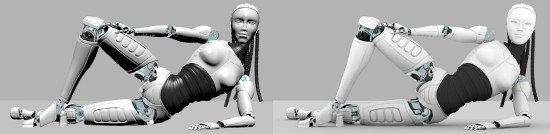Left image rendered with standard diffuse lighting. Right image rendered with ambient occlusion.

## Introduction

When light bounces off a surface, it contributes to the amount of ambient lighting, or environment lighting we see. In a basic renderer, the amount of ambient lighting a surface receives is uniform, making it look rather flat. Ambient occlusion provides a more realistic shading technique by taking into account that nearby objects may occlude the amount of ambient light reaching a surface. The closer and/or larger an occluder is to the surface being tested, the more shaded the surface becomes. This added shading technique provides greater depth visualization, thus improving the overall image quality. This article will cover the general ambient occlusion technique used in ray tracing and how it can be used in a hardware accelerated approximation technique called SSAO.

## Ambient Occlusion

Ambient occlusion calculates the amount of ambient lighting a surface receives by taking into account that objects nearby may block some of the ambient lighting that reaches the surface. It works by casting out rays up to 90 degrees from the normal of the surface being tested. If any of these rays hit another surface, then an attenuated diffuse term is calculated and summed to the overall amount of occlusion that surface receives.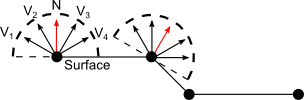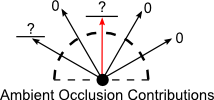The formula for calculating the ambient occlusion contributions:

$AO = \frac{\sum\limits_{i=1}^n (max((\vec{N} \cdot \vec{V_i}), 0.0) * A)}{n}$ $A = \frac{1.0}{(A_C + A_L + A_K)}$ $A_L = A_l * d$ $A_K = A_k * d^2$

Where

$n$ is the number of samples to calculate.

$\vec{N}$ is the vertex normal.

$\vec{V_i}$ is a vector from the vertex to the occluder's surface (0 if there is no occluder).

$A_C$ is an attenuation constant.

$A_L$ is the calculated linear attenuation and $A_l$ is the linear constant used in that calculation.

$A_K$ is the calculated quadratic attenuation and $A_k$ is the quadratic constant used in that calculation.

$d$ is the distance, or length of the vector $V_i$.

$A$ is the calculated attenuation factor that will multiply the occlusion factor.

The dot product in this equation ensures that occluders at an angle to the testing surface add smaller contributions than occluders directly above the surface. This is similar to the diffuse lighting where a surface normal pointing toward a light source receives greatest illumination compared to a surface where the light source is at its horizon. The attenuation factor ensures that occluders nearby contribute more than occluders further away. As you bring two surfaces together, the amount of ambient lighting decreases, thus increasing the amount of shading.

## SSAO

Ambient occlusion requires that each point on a surface cast out several rays to sample the ambient occlusion contributions. The more rays you cast, the smoother and more accurate your result. This is computationally expensive for real-time graphics. Instead, AO calculations are performed in screen space by sampling neighbouring pixels. This requires you setup a deferred rendering system where the positions (vertices), normals, and colours are stored into texture buffers and post-processed in an SSAO shader.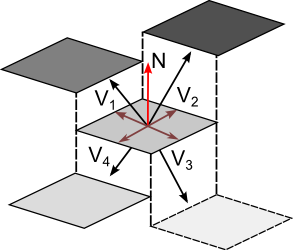Screen space AO illustration using an isometric view of the framebuffer. Neighbouring pixels are sampled and their 3D view-space positions will either be in front or behind the pixel being tested. V1 and V2 will provide AO contributions as they are in front, but V3 and V4 are behind and are therefore excluded.

### Prerequisits

Deferred rendering requires that you store your geometry data using floating point textures. WebGL and OpenGL ES 2.0 do not natively support this texture format; however WebGL introduced an extension called "OES_texture_float" to enable floating point texture support. To enable this extension, you must call the function gl.getExtension("OES_texture_float"). This function will return null if the extension failed to load or non-null if the extension successfully loaded. Once the extension is enabled, you can create floating point textures in your framebuffer objects simply by creating 2D textures with the type set to gl.FLOAT instead of gl.BYTE.

WebGL and OpenGL ES 2.0 also don't support multiple colour attachments to the framebuffer object. Due to this limitation, you must render your geometry data multiple times to different framebuffer objects. This is slightly slower compared to doing everything in one pass, which would be possible in standard OpenGL and supported hardware.

### Step 1: Colour buffer

Render the scene with normal transform and lighting to a standard RGB texture. This will be used later to blend the AO contributions.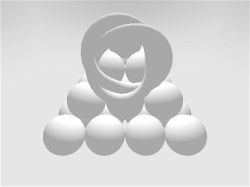Visualization of the colour buffer.

### Step 2: Position buffer

Render the 3D view-space positions to a floating point texture.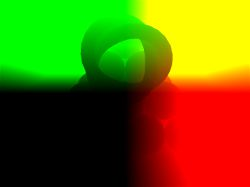Visualization of the position buffer.

### Step 3: Normal buffer

Render the 3D view-space normal vectors to a floating point texture.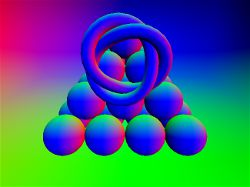Visualization of the normal buffer.

### Step 4: SSAO Contributions

Supply the position texture, normal texture, and optionally a random normalmap to the SSAO shader. These three will be used to calculate the ambient occlusion contributions for each fragment. Since the sampling size is kept small for performance reasons, the normalmap is used to perturb the sampling kernel to produce a more noisy solution. If you skip this step, your sampling will produce a noticeable banding effect.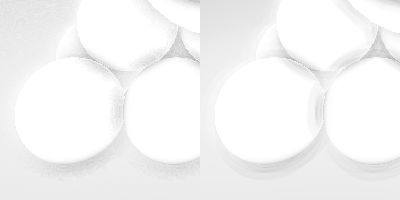The image on the left uses a perturbed sampling kernel that produces a noisy, but uniform result. The image on the right uses a simple sampling kernel, which produces a banding effect due to a small sampling size.

The first step is to produce the sampling kernels. For sampling 16 pixels, you produce a set of 4 kernels, each sampling 4 pixels and sequentially rotated 45 degrees as the kernels expand outwards from the centre.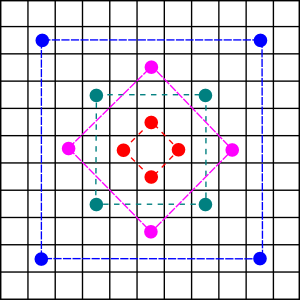Illustration of the 4 sampling kernels.

You then perturb the kernels using a high frequency normalmap. You can calculate the perturbed kernel vector by reflecting it with the 2D vector stored in the normalmap (you can discard the z-axis in this case) . This random rotation in the kernel will add high frequency noise to the final result. It's important to use a high frequency normalmap to prevent the SSAO shader from producing visual patterns in the final result, similar to rendering perlin noise on the screen.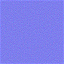Example 64x64 high frequency normalmap.

The size of your sampling kernel should depend on the distance from the camera. Pixels at the back of the framebuffer should have a smaller kernel then those up front. When rendering the geometry's 3D view-space position data to a texture, you should include its linear depth value in the alpha channel. This can be used to adjust the size of the sampling kernel based on its distance from the camera.

$Kernel\_Radius = Radius * (1.0 - Linear\_Depth)$

Fragments furthest back will have a linear depth closer to 1.0, thus reducing the radius to 0. Fragments close up will have a linear depth closer to 0, producing a maximum sampling radius.

Now use the AO formula, sum the AO contributions from each sampled pixel.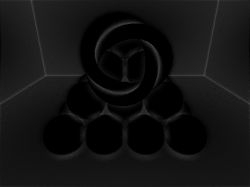Visualization of the AO buffer. Black regions represent no occlusion, whiter regions represent occluded, or shaded areas.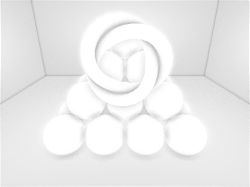Visualization of the inverted AO buffer.

### Step 5: Blending the results

The final step is to blend the colour buffer with the AO buffer. Since the black regions represent no occlusion and the whiter regions represent occlusion, or shaded areas, you simply subtract the AO buffer from the colour buffer and clamp the results between 0.0 and 1.0.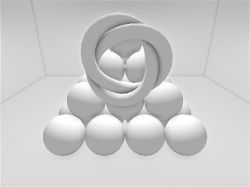Comparison of renders before and after AO is applied.

## Problems

SSAO can improve image quality, but it's not without its problems.

### View Dependence

SSAO is a view dependent algorithm. When you transform your view, you are effectively changing the data that gets calculated. Pixels that were not seen before may be visible at a certain angle, possibly adding to the overall AO contribution a pixel receives and thus changing the result.

### Noise

Using a randomized kernel produces a noisy, but uniform result. To remove this noise, you need to blur the AO map using a separable blur algorithm. However, you cannot just blur the AO map like you would a glowmap or any other such texture. You do not want to bleed the results into neighbouring pixels that should not be shaded, so you must implement a specific blurring shader that blends only pixels with AO applied. Blurring noisy textures also produces smudging, which makes the texture look watered down and thus reduces the overall image quality. It can be difficult to find a satisfactory balance. Good use of texturing can help eliminate any visible noise.

If your attenuation factor is small, pixels at further distances will be included in the overall AO contributions, creating false shading.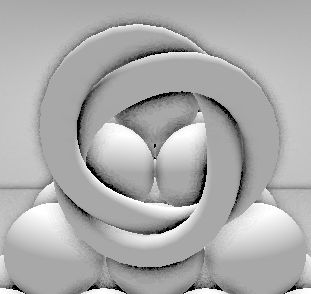You can see the dark shading surrounding the torus knot due to using such a low attenuation factor. The wall in the back thinks the torus knot in front is close enough to shade it. To resolve this issue, you should use a sufficiently large attenuation factor so that distant surfaces get factored out. Increasing your attenuation factor can also brighten your AO results, thus making it harder to see any shading. You need to find the right balance and what works best for your scenes.

### Self Occlusion

When computing the dot product, it's possible that the surface may end up sampling from the same triangle or quad. This can cause edges to appear as shaded regions, giving a wireframe look. To resolve this issue, you should apply a bias to the dot product that will discard any fragments close to the horizon.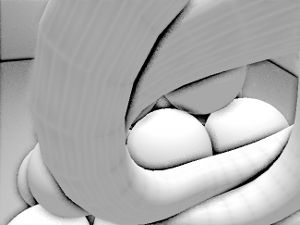$AO = \frac{\sum\limits_{i=1}^n (max((\vec{N} \cdot \vec{V_i}) - bias, 0.0) * A)}{n}$

The revised formula applies a bias to the dot product to minimize self occlusion. A bias of 0.05 works well. Using a large bias will decrease the amount of AO shading that is calculated. You need to find the right balance if you have geometry that's difficult to work with.

## Conclusion

Despite some of its problems, ambient occlusion greatly improves image quality. Like all visual improvements however, care should be taken to allow users to opt out of this effect. 16 samples per pixel, floating point textures, deferred rendering passes are all quite expensive and can create bottlenecks when combined with other rich features such as shadow mapping, depth of field, HDR rendering, etc. Nevertheless, ambient occlusion works well with WebGL and allows for richer looking online games.

The source code for this project is made freely available for download. The ZIP package below contains both the HTML and JavaScript files to replicate this WebGL demo.

The source code utilizes the Nutty Open WebGL Framework, which is an open sourced, simplified version of the closed source Nutty WebGL Framework. It is released under a modified MIT license, so you are free to use if for personal and commercial purposes.